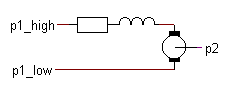﻿ 20-sim webhelp > Library > Iconic Diagrams > Electric > Actuators > dcmotor

dcmotor

Library

Iconic Diagrams\Electric\Actuators

Iconic Diagrams\Mechanical\Electric

Default

IR

Use

Domains: Continuous. Size: 1-D. Kind: Iconic Diagrams (Electric, Electric).

Description - Default

This models represents an ideal DC-motor with no energy loss. The electric port has separate high and low terminals. The equations are

p1.i = p1_high.i = p1_low.i;

p1.u = p1_high.u - p1_low.u;

The model can have mixed forms of causality

p1.u = k * p2.omega;

p2.T = k * p1.i;

or:

p2.omega = p1.u / k;

p1.i = p2.T / k;

Interface - Default

 Ports Description p1_high, p1_low p2 Both terminals of the Electric port p1. Rotation port. Causality mixed See equations above. Parameters k motor constant [Nm/A]

Description - IR

This models represents an ideal DC-motor with inductance and resistance.The electric port has separate high and low terminals. The equations are

p1.i = p1_high.i = p1_low.i;

p1.u = p1_high.u - p1_low.u;

Interface - IR

 Ports Description p1_high, p1_low p2 Both terminals of the Electric port p1. Rotation port. Causality mixed See equations above. Parameters k L R motor constant [Nm/A] motor inductance [H] motor resistance [Ohm]